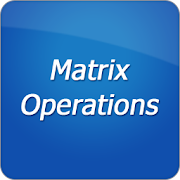# Matrix Operations - Lumos Educational App Store4.11
Price -fREE
\$0

#### DESCRIPTION:

The application lets you to perform some simple operations and calculations with matrices: - Transposition - Calculate determinant - Sum and diff of matrices - Multiplication of matrices - Multiplication of a matrix by number - Calculate rank - Find inverse - Solve systems of linear equations (with Gauss algorithm) A*X=B.

#### OVERVIEW:

Matrix Operations is a free educational mobile app By .It helps students in grades HS practice the following standards HSN.VM.C.6.

This page not only allows students and teachers download Matrix Operations but also find engaging Sample Questions, Videos, Pins, Worksheets, Books related to the following topics.

1. HSN.VM.C.6 : Use matrices to represent and manipulate data, e.g., to represent payoffs or incidence relationships in a network..

HS

#### STANDARDS:

HSN.VM.C.6

Developer:

Software Version: 2.08

Category: Education

### RELATED APPSEdSearch WebSearch